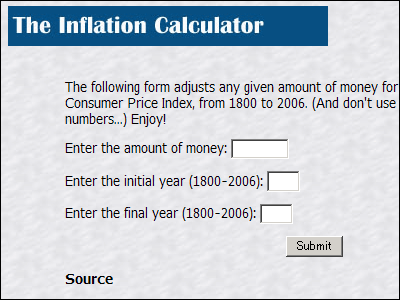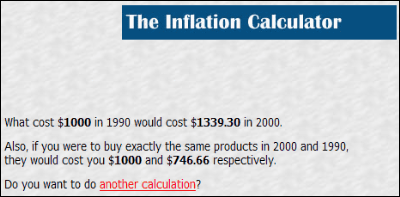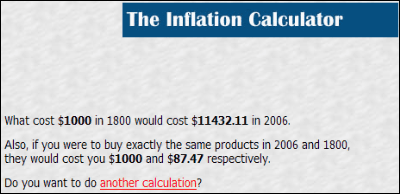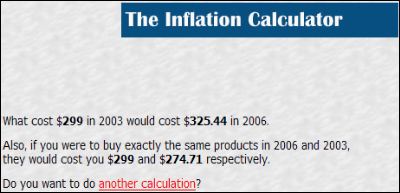# "The Inflation Calculator" that can calculate the value of dollars for any yearWhen you enter any two years and amounts (dollars), it is an inflation calculator that tells you how much the value changes in two years. We use the consumer price index and can calculate the age from 1800 to 2006. For example, you can know how much it is equivalent to \$ 1,000 in 1990 in 2006.

Access is from the following.
The Inflation Calculator

Just enter the amount in the top frame, year into the next two and press Submit.

For example, 1000 dollars in 1990 corresponds to 1,339 dollars and 30 cents in 2000. So for 1000 dollars in 2000 it will be 746 dollars 66 cents if it is 1990.If you calculate it the furthest, 1,000 dollars in 1800 will be 11,142 dollars and 11 cents in 2006. In fact it is more than 10 times.Think about it with specific products.Worldwide sales volume of handheld game machines Ranking worst 10Nokia's N-Gage that appeared on the market was released for \$ 299. It seems that this is now 325 dollars and 44 cents. Then, the Japanese yen equivalent is not about 35,000 yen, it is about 38,800 yen, and the feeling of more expensive is increased.It may be interesting to look at where elongation is putting in various ages.

in Web Service, Posted by logc_nt